# Le Chatelier's Principle

This is part of the HSC Chemistry course under the topic Factors that Affect Equilibrium

### HSC Chemistry Syllabus

• Investigate the effects of temperature, concentration, volume and/or pressure on a system at equilibrium and explain how Le Chatelier’s principle can be used to predict such effects, for example:

– heating cobalt (II) chloride hydrate

– interaction between nitrogen dioxide and dinitrogen tetroxide

– iron(III) thiocyanate and varying concentration of ions (ACSCH095)

• Explain the overall observations about equilibrium in terms of the collision theory (ACSCH094)

• Examine how activation energy and heat of reaction affect the position of equilibrium

### What is Le Chatelier's Principle and how does it apply to equilibria?

This video will explore what Le Chatelier's Principle is and discuss its relevance regarding understanding how equilibrium positions are manipulated.

### What is Le Chatelier's Principle?

• Le Chatelier’s principle predicts the outcome of a reversible chemical system when a change is imposed on it. It states:

“If a change is imposed on a system at equilibrium, the position of the
equilibrium will shift in a direction that tends to reduce that change.”

### Concentration

• Concentration directly influences the rate of collision between molecules and atoms which in turn affects the rate of reaction.Figure: addition of a chemical increases its concentration and rate of collision with other molecules which in turn increases the reaction rate. This disturbs the equilibrium as rates of forward and reverse reactions are no longer equal.

• When concentration of reactant(s) increases:
1. The forward reaction rate will increase due to greater rate of particle collision.
2. By Le Chatelier’s principle, the system will act to consume some of the reactants to reduce the rate of collision and also the forward reaction rate.
3. Concentration of product will increase which increases the rate of reverse reaction.
4. Equilibrium is reached when forward reaction rate equals reverse reaction rate.

• When concentration of reactant(s) decreases:
1. The forward reaction rate will decrease due to lower rate of particle collision.
2. By Le Chatelier’s principle, the system will act to consume some of the products to reduce the rate of collision and thus the reverse reaction rate.
3. Concentration of reactants will increase which increases the rate of forward reaction.
4. Equilibrium is reached when forward reaction rate equals reverse reaction rate.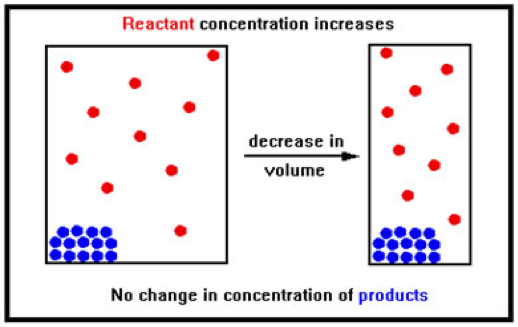Figure: r
eduction in volume increases pressure on gases and the collision rate between them. Solids and liquids are not affected by changes in pressure and volume (the effect is negligible compared to gases).

### Pressure/Volume

• Pressure and volume affect the collision rate between gas molecules. The effect on aqueous, liquid and solid-state compounds and molecules is negligible, thus it is generally disregarded.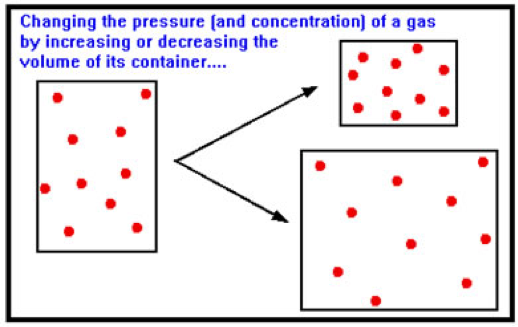Figure: changes in pressure or volume directly impact the collision rate and thus, the rate of reaction. An increase in pressure increases reaction rate while a decrease in pressure decreases reaction rate.

• Changes in pressure and volume have greater effect on collision rate and reaction rate when there are more molecules present.

1. An increase in pressure (decrease in volume) increases the rate of collision between molecules (for both reactant and products).
2. However, the increase would be greater for the side that has more moles of gas.
3. This causes a net increase in molecules on the side with less moles of gas. In other words, the equilibrium position will shift to the side with less moles of gas when pressure is increased.

• For example, the dynamic equilibrium between nitrogen dioxide and dinitrogen tetroxide:

$$2NO_{2(g)} \rightleftharpoons N_2O_{4(g)}$$

• The forward reaction consumes 2 molecules of NO2 to produce 1 molecule of N2O4.Figure: changes in pressure and volume have greater effect when there are more gas molecules. In this example, there are more reactants (blue), so changes in pressure exert greater effects on the forward reaction rate.

• An increase in pressure (decrease in volume) increases the rate of the forward reaction more than the reverse. This shifts the chemical equilibrium towards the right side to produce more . Number of gas molecules present in the system is reduced.
• A decrease in pressure (increase in volume) decreases the rate of forward reaction more than the reverse. This shifts the chemical equilibrium towards the left side to produce more NO2 and increase entropy.

Example: The graph below is plotted for a reversible reaction.

$$2A_{(g)}+B_{2(g)} \rightleftharpoons 2AB_{(g)}$$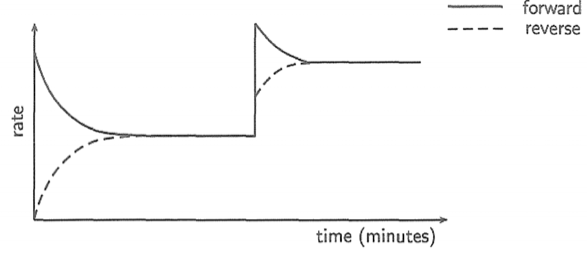What change has been imposed on the system?

The reaction reached and remained in equilibrium for a while until a change has been imposed, increasing rates of forward and reverse reactions.

The pressure of the system has increased. This increases the rate of both forward and reverse reaction. However, since the forward reaction has greater number of gas molecules, the forward reaction rate increases more.

By Le Chatelier’s principle, the system will consume some reactants to form products. This reduces the forward reaction rate while increases the reverse reaction rate.

### Temperature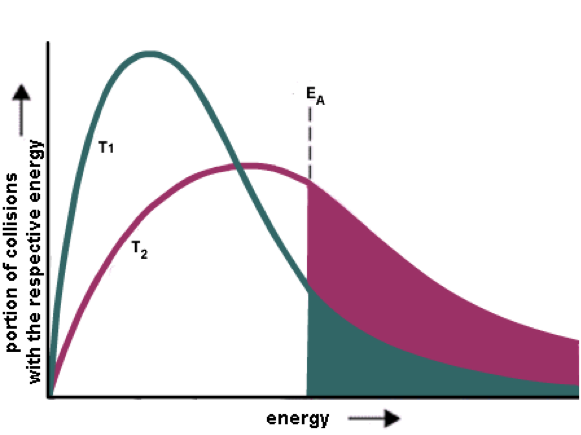Figure: A rise in temperature causes more particles to have energy greater than the activation energy. This means reaction rate is greater due to more successful collisions. T2 > T1.

• In collision theory, temperature affects rate of collision as well as the energy of molecules during collisions. Higher temperature means there is more energy in the system and therefore, more molecules will have energy greater than the activation barrier. This increases the rate of reaction.
• Changes in temperature affect the rate of both forward and reverse reactions. However, it has a greater impact on the reaction with a larger activation energy (Ea).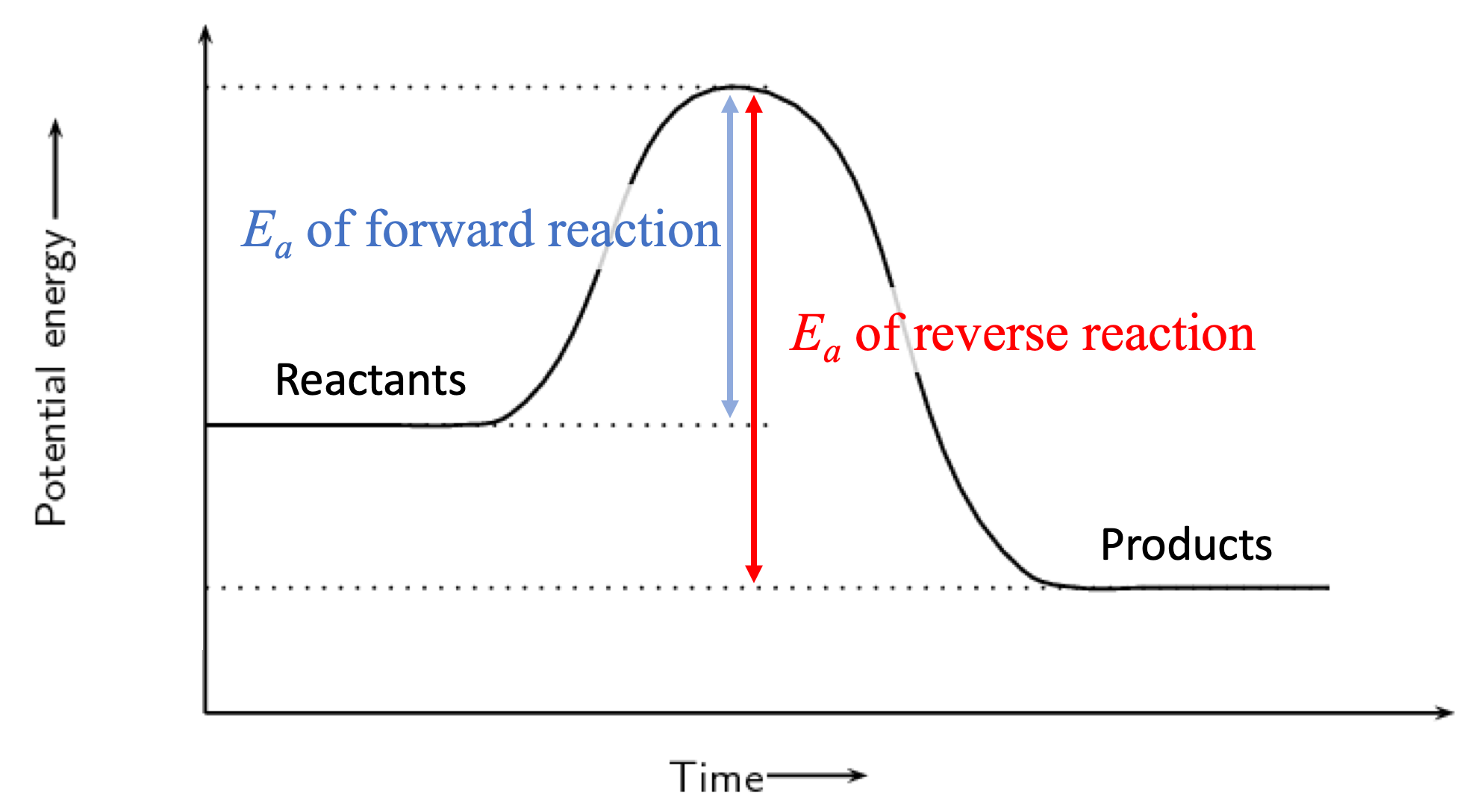Figure: energy profile of an exothermic reaction. The reverse reaction is endothermic and has a larger activation energy barrier than the forward reaction.

• The effect of temperature on a reaction at chemical equilibrium depends on the nature of its enthalpy – endothermic or exothermic. If the forward reaction is endothermic, then the reverse reaction is exothermic.
• If forward reaction is endothermic (DeltaH>0), an increase in temperature favours the forward reaction more because it has a larger activation energy than the reverse reaction.
• If forward reaction is exothermic (DeltaH<0), an increase in temperature favours the reverse reaction. Conversely, a decrease in temperature favours the forward reaction.
• For example, the dynamic equilibrium between nitrogen dioxide and dinitrogen tetroxide:

$$2NO_{2(g)} \rightleftharpoons N_2O_{4(g)} \;\;\; \Delta H=-58 \; kJ mol^{-1}$$

The forward reaction is exothermic, so an increase in temperature will favour the reverse reaction rate more (endothermic). Conversely, when temperature decreases, the forward reaction is favoured more (exothermic).

### CatalystFigure: catalysts reduce the activation energy of both forward and reverse reactions.

• Addition of catalyst(s) increases the rate of both forward and reverse reactions by reducing their respective activation energies.

Catalysts do not disturb chemical equilibriaFigure: addition of catalyst at the 10-minute mark increases the rate of both forward and reverse reaction. The equilibrium is not disturbed.

• Catalysts increase forward and reverse reaction rates to the same extent. As a result, they do not affect the equilibrium constant nor the position of equilibrium.

Reactions reach equilibrium faster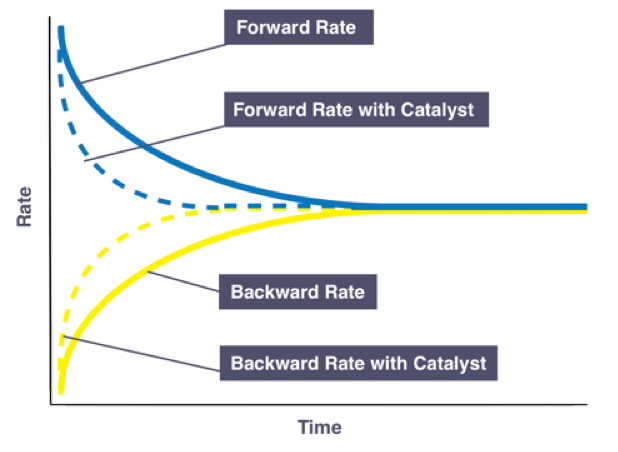Figure: addition of a catalyst allows the equilibrium to be reached faster.

• In the presence of a catalyst, a reversible reaction reaches equilibrium faster.

## Practice Question 1

The following graph shows the change in reaction rate with time for the following generic reaction.
$$2AB_{(g)} \rightleftharpoons 2A_{(g)} + B_{2(g)}\;\;\; \Delta{H}>0$$What change is happening in this question? Why cannot it not be a change in pressure?

## Practice Question 2

Industrial synthesis of ammonia uses nitrogen gas and hydrogen gas as reactants. The chemical equation for this process is:(a) What is happening at t1? How many chemical species change in concentration instantaneously?

(b) What is happening at t2? How many chemical species change in concentration instantaneously?

(c) What is happening at t3? Is the change in concentration instantaneous?

## Practice Question Solution

Practice Question 1

$$2AB_{(g)} \rightleftharpoons 2A_{(g)} + B_{2(g)}\;\;\; \Delta{H}>0$$There are two possible changes that would have caused an increase in rate of forward and reverse reaction: (1) temperature and (2) pressure/volume. Since the reaction rates have increased, the change must be either an increase in temperature or increase in pressure (reduced volume).

The forward reaction is endothermic so an increase in temperature would increase the forward reaction rate more than the reverse reaction rate. This is shown in the graph as, after the change, forward reaction rate is higher than the reverse reaction rate. Since equilibrium is disturbed, rates of forward and reverse reactions subsequently decreased and increased respectively to re-achieve equilibrium.

Why is the change not pressure?

The answer in this case cannot be an increase in pressure as there are more gas molecules on the product side than the reactant side. There are 3 moles of gases on the product side for every 2 moles on the reactant side. This means an increase in pressure would have increased the reverse reaction (3 moles of gases) more than the forward reaction (2 moles of gases). This was not observed in the graph.

Practice Question 2

$$N_{2(g)}+3H_{2(g)} \rightleftharpoons 2NH_{3(g)}\;\;\; \Delta{H} = -92\, kJmol^{-1}$$Before beginning the question, it is important to note that this is not a rate versus time graph but instead it is a concentration versus time graph.

(a) At t_1, only [N_2] increased instantaneously which suggests this is not a change in pressure/volume as it would otherwise affect concentrations of all chemical species in the reaction. This is also not a change in temperature as changes in temperature do not change concentrations of species instantaneously. Therefore, the change at t_1 is likely to be addition of nitrogen gas.

(b) At t_2, concentrations of all chemical species of the reaction have increased instantaneously. This suggests an increase in pressure on the system (or reduction in volume of vessel). This can be confirmed by examining the equation of the reaction:

$$N_{2(g)}+3H_{2(g)} \rightleftharpoons 2NH_{3(g)}\;\;\; \Delta{H} = -92\, kJmol^{-1}$$

Since there are more moles of gases on the reactant (left) side, rate of forward reaction is favoured by an increase in pressure. This causes greater quantities of NH_3 (ammonia) to be produced, which is shown as a gradual change right after the pressure change at t_2.

(c) No chemical species at have changed their concentrations instantaneously at t_3. This means the change is associated with temperature. The concentrations of N_2 and H_2 gradually increased, and that of NH_3 decreased. This indicates that the temperature change favours the reverse reaction. Since the reverse reaction is endothermic, the change must an increase in temperature.

Previous section: Collision Theory in Equilibria